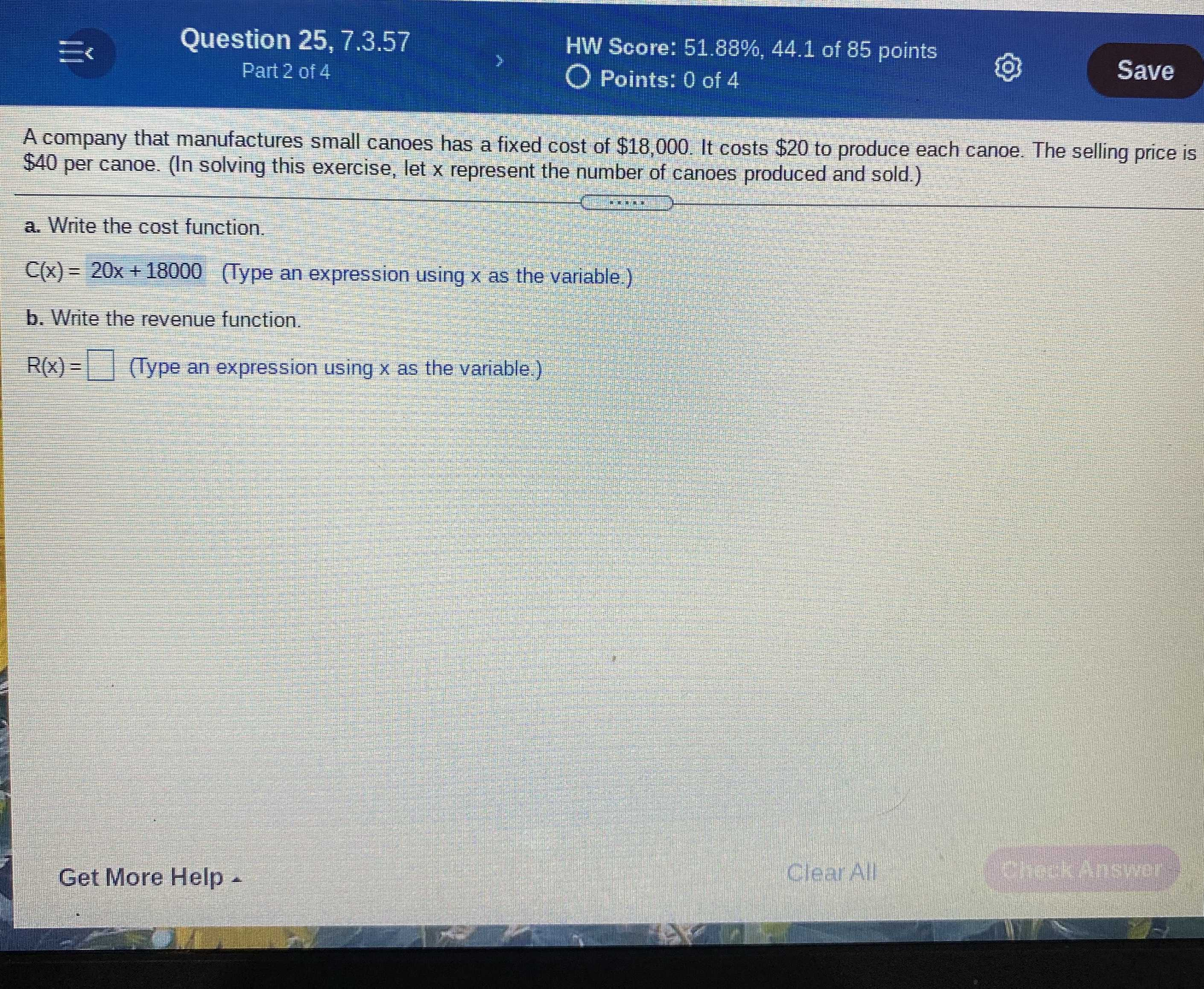### ¿Todavía tienes preguntas de matemáticas?

Pregunte a nuestros tutores expertos
Algebra
PreguntaA company that manufactures small canoes has a fixed cost of $$\ 18,000$$ . It costs $$\ 20$$ to produce each canoe. The selling price is $$\ 40$$ per canoe. (In solving this exercise, let $$x$$ represent the number of canoes produced and sold.) a. Write the cost function. $$C ( x ) = 20 x + 18000$$ (Type an expression using $$x$$ as the variable.) b. Write the revenue function. $$R ( x ) = \square$$ (Type an expression using $$x$$ as the variable.)